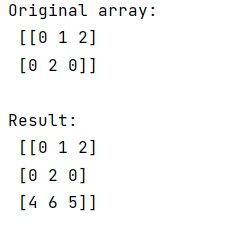# Add row to a NumPy array

Learn, how to add row to a NumPy array?
Submitted by Pranit Sharma, on December 21, 2022

NumPy is an abbreviated form of Numerical Python. It is used for different types of scientific operations in python. Numpy is a vast library in python which is used for almost every kind of scientific or mathematical operation. It is itself an array which is a collection of various methods and functions for processing the arrays.

## Adding row to a NumPy array

Rows in NumPy are the different values that are aligned horizontally and also provide uniformity. Each row can have the same or different value. Rows are generally marked with the index number.

Suppose we are given a NumPy array with some existing rows and we need to add rows to this array from another array by satisfying a condition that if the first element of each row in the second array is less than a specific value.

To add a new row, we will use the numpy.append() method where we will pass the NumPy array which we want to add as a row.

But since we need to satisfy a condition, we will loop over the second array and check whether each of its elements that it satisfies the condition or not. If it satisfies the condition, we will append this value as an element of a new row in the original array.

Let us understand with the help of an example,

## Python code to add row to a NumPy array

```# Import numpy
import numpy as np

# Creating a numpy array
arr = np.array([[0, 1, 2], [0, 2, 0]])

# Display original array
print("Original array:\n",arr,"\n")

# Creating another array
x = [4,6,2,3,5]

# Creating an  empty list
l = []

# Looping over second array and
# checking the condition
for i in x:
if i>=4:
l.append(i)

# Appending list to row
arr = np.append(arr,l)

# Display Result
print("Result:\n",arr)
```

Output: